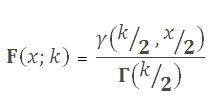# SQL Server CHISQ.INV function

Updated: 9 August 2010

Use CHIINV to calculate the inverse of the one-tailed probability of the chi-square distribution. The chi-square distribution is associated with a chi-square result. The chi-square distribution is a special case of the gamma distribution. Its cumulative distribution function is:Syntax
SELECT [wctStatistics].[wct].[CHIINV] (
<@Probability, float,>
,<@Degrees_freedom, float,>)
Arguments
@Probability
is any number between zero and one. @Probability is an expression of type float or of a type that can be implicitly converted to float
@Degrees_freedom
is any real positive number. @Degrees_freedom is an expression of type float or of a type that can be implicitly converted to float

Return Types
float
Remarks
·         If @Probability < 0 or @Probability> 1, CHIINV returns an error.
·         @Degrees_freedom is truncated to zero decimal places.
·         if @Degrees_freedom < 1 or @Degrees_freedom > 10^10, CHIINV returns an error.
·         CHIINV uses root-finding algorithms and iteration to solve for CHIDIST(CHIINV(@Probability, @Degrees_freedom),@Degrees_freedom)-@Probability = 0 to eight decimal places
·         CHIINV = GAMMAINV(1-@Probability, @Degrees_freedom/2, 2)
Examples

select
wct.CHIINV(.01, 5)

This produces the following result

----------------------
15.0862724691398

(1 row(s) affected)

select round(wct.CHIDIST(wct.CHIINV(.01, 5),5)-.01, 8)

This produces the following result

----------------------
0

(1 row(s) affected)

Select wct.GAMMAINV(1-.01,2.5,2)

This produces the following result

----------------------
15.0862724691401

(1 row(s) affected)

### SupportCopyright 2008-2021 Westclintech LLC         Privacy Policy        Terms of Service